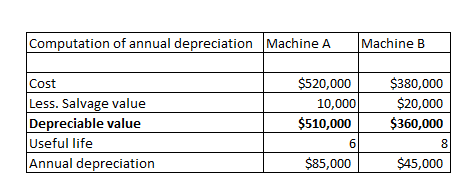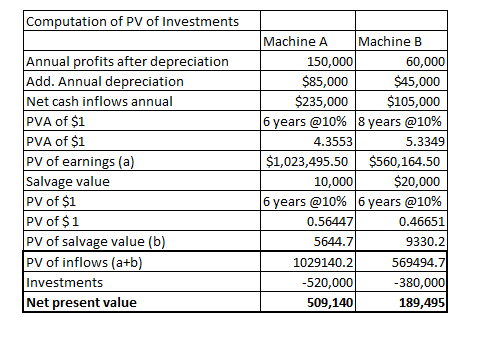# a. A new operating system for an existing machine is expected to cost \$520,000 and have a useful life of six years. The system yields an incremental after-tax income of \$150,000 each year after deducting its straight-line depreciation. The predicted salvage value of the system is \$10,000.b. A machine costs \$380,000, has a \$20,000 salvage value, is expected to last eight years, and will generate an after-tax income of \$60,000 per year after straight-line depreciation.  Assume the company requires a 10% rate of return on its investments. Compute the net present value of each potential investment. (PV of \$1, FV of \$1, PVA of \$1, and FVA of \$1) (Use appropriate factor(s) from the tables provided.)

Question
235 views

a. A new operating system for an existing machine is expected to cost \$520,000 and have a useful life of six years. The system yields an incremental after-tax income of \$150,000 each year after deducting its straight-line depreciation. The predicted salvage value of the system is \$10,000.
b. A machine costs \$380,000, has a \$20,000 salvage value, is expected to last eight years, and will generate an after-tax income of \$60,000 per year after straight-line depreciation.

Assume the company requires a 10% rate of return on its investments. Compute the net present value of each potential investment. (PV of \$1, FV of \$1, PVA of \$1, and FVA of \$1) (Use appropriate factor(s) from the tables provided.)

check_circle

Step 1

We need to add back annual depreciation to after tac profits, so that we are able to determine the cash inflows. It is to be noted that depreciation is a non-cash item.

To determine the Net present value of investments, we will determine the net present value of annuities (annual cash flows) add salvage value of machine and subtract the initial investments

Step 2...

### Want to see the full answer?

See Solution

#### Want to see this answer and more?

Solutions are written by subject experts who are available 24/7. Questions are typically answered within 1 hour.*

See Solution
*Response times may vary by subject and question.
Tagged in